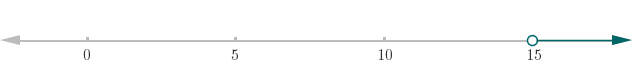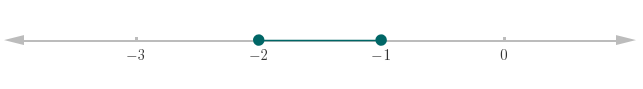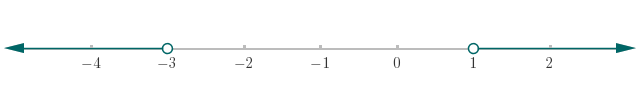Inequalities homework Help at TutorEye

## Top Questions

E.g. 5% charge on every 10% breach of the total breach of 102%. Any short summed-up formula?
View More

How far did he run on Tuesday? Enter your answer in the box as a fraction in simplest form.
View More

ou’ll be using the same 4 numbers written in the same order. The only thing that will change in each expression will be the tools you’ll use from your toolbox. The tools that you’ve learned so far for order of operations are parentheses, exponents, multiplying, dividing, adding, and subtracting. Here are the four numbers. Keep them in this order: 18 2 4 3 Here are the tools in your toolbox: The 2 and 3 represent exponents, so you are allowed to square or cube a number. Listed below are the values you need to create. 21 = 67 = -6 = 103 =
View More

profit of 20%. If his gain on the sale of one electronic item is rupees 4500 find the Marked price of the electronic item.
View More

## Inequalities Sample Questions

Question 1: Solve for unknown variable x, and write the answer using interval notation.Answer:Explanation:  Expand the bracket. And, rearrange the terms to solve forQuestion 2: Solve for q.Answer:Explanation:  Rearrange the terms to solve for q.

Question 3: Solve for r, and write the solution using interval notation.Answer:Explanation:  Expand the bracket. And, rewrite the terms to solve for.

Question 4: Solve for unknown variable x, and write the solution using interval notation.Answer:Explanation:  Subtract 2 from all parts of inequality.

Question 5: Solve for unknown variable x, and write the solution using interval notation.Answer:Explanation:  Add 3 to all parts of inequality.

Question 6: Solve for y.Answer:Explanation:  Add 3 to both sides of inequality.

Question 7: Solve for z, and write the answer using interval notation.Answer:Explanation:  Rearrange the terms to isolate the z.

Question 8: Solve for a. And, show the solution on the number line.Answer:Explanation:  Rearrange the terms to isolate the a.

Question 9: Solve for b. And, show the solution on the number line.Answer:Explanation:  Add 4 to all sides of the inequality.

Question 10: Solve for x, write the interval solution. And, show the solution on the number line.orAnswer:Explanation:  Solve each inequality.